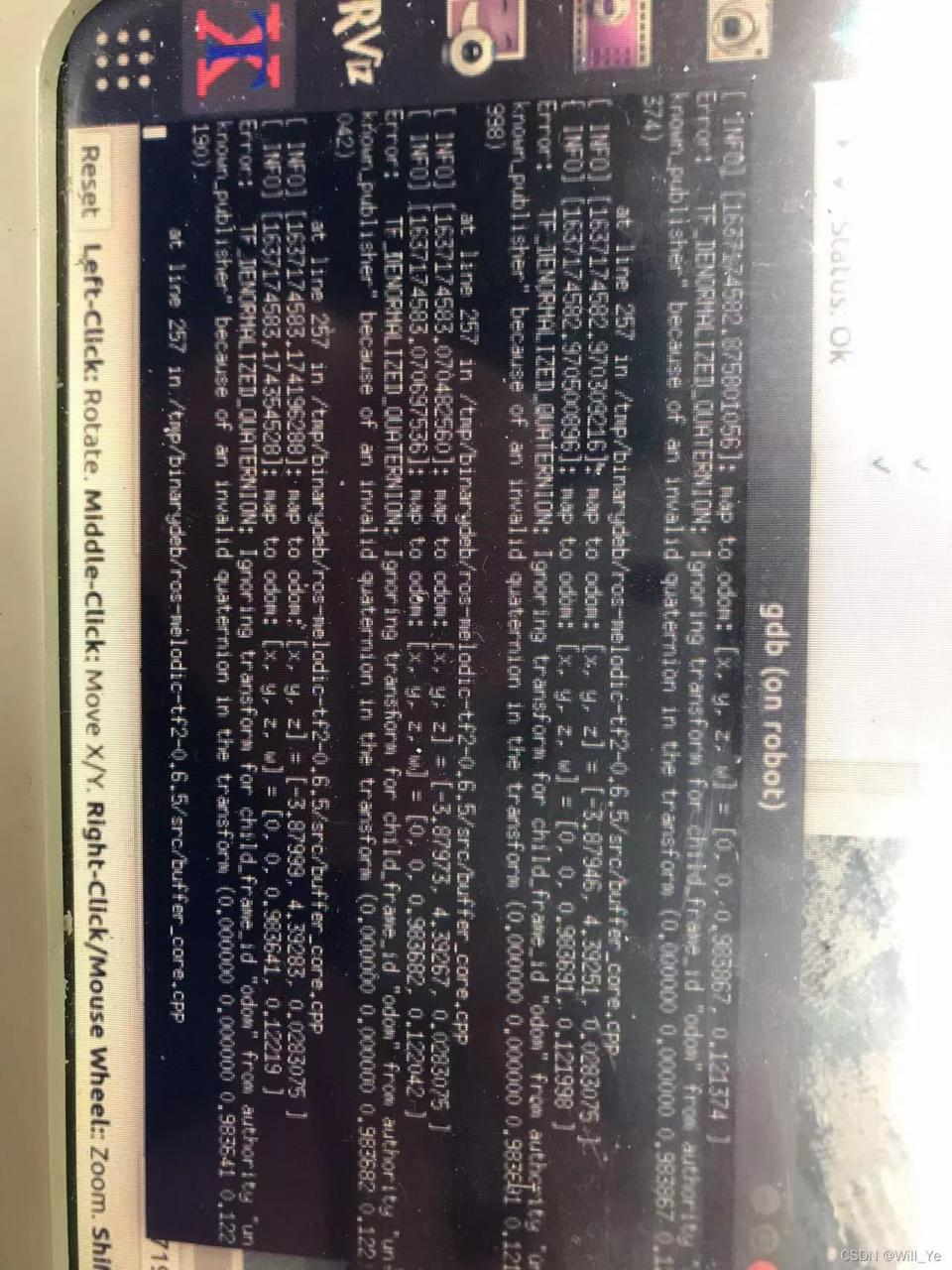# TF Error: Error: TF_DENORMALIZED_QUATERNION [How to Solve]

1. Problem

HDL locationing Error:

Error: TF_DENORMALIZED_QUATERNION: Ignoring transform for
child_frame_id “odom” from authority “unknown_publisher” because of an
invalid quaternion in the transform (0.0 0.0 0.983641 0.122190)2. Solution

From the error report can be seen, the tip is a quadratic number of problems, the key is to say that there is no normalization, the release of TF has strict requirements, the quadratic number must be normalized, that is, xyzw squared and equal to 1, if not equal to the error reported above, a simple calculation, the above error tip quadratic number is: (0.0 0.0 0.983641 0.122190), that is, 0.98364 1 2 + 0.12219 0 2 = 0.982480012981 0.983641^2 + 0.122190 ^2 = 0.9824800129810.983641^2 + 0.122190^2 = 0.982480012981, obviously not equal to 1, so there will be the above problem, the solution is also simple, before the output Do a normalization process can be.

``````         Eigen::Quaterniond quatern = {0.0 0.0 0.983641 0.122190}; // w x y z
quatern.normalize();
odom_trans.transform.rotation.x = quatern.x();
odom_trans.transform.rotation.y = quatern.y();
odom_trans.transform.rotation.z = quatern.z();
odom_trans.transform.rotation.w = quatern.w();